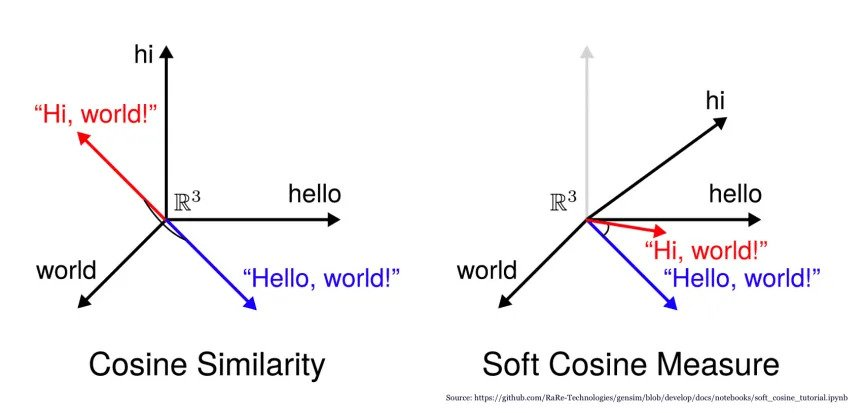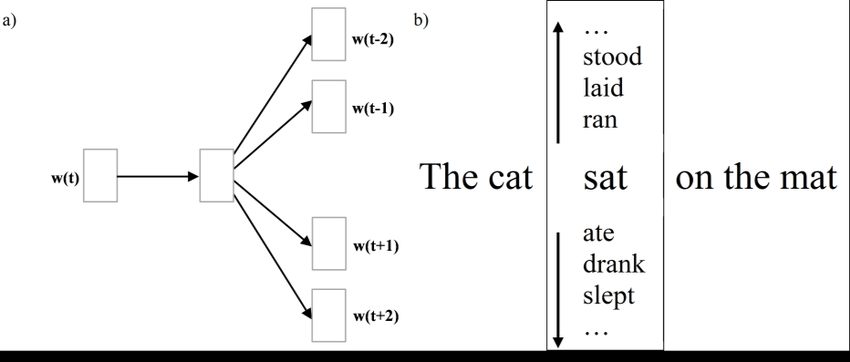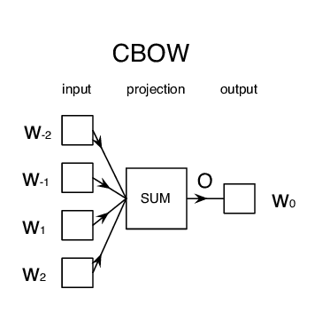×

Search anything:

# Word Embedding [Complete Guide]

#### Natural Language Processing (NLP) Machine Learning (ML)Get this book -> Problems on Array: For Interviews and Competitive Programming

In this article, we have explained the idea behind Word Embedding, why it is important, different Word Embedding algorithms like Embedding layers, word2Vec and other algorithms.

1. Introduction to Word Embedding
2. Is Word Embedding Important?
3. Word Embedding Algorithms
3.1. Embedding Layer
3.2. Word2Vec
3.2.1. Skip-Gram
3.2.2. Continuous Bag of Words (CBOW)
3.3. GloVe
4. Conclusion

Let us get started.

# Introduction to Word Embedding

Maybe some of you that read this article have a basic knowledge about Machine Learning and how to use it. Machine Learning learns from datasets with table formats that built up by using numbers. But in this world, not all things made up from numbers. How about we have a data that built up from words and sentences, not from numbers? To solve that particular problem, we have NLP or Natural Language Processing. NLP only transform words into sets of numbers, and what if words have similar or same meaning?

Word Embedding is one of the most popular representation of document vocabulary. It is capable of capturing context of a word in a document, semantic and syntactic similarity, relation with other words, etc.

Word embeddings are in fact a class of techniques where individual words are represented as real-valued vectors in a predefined vector space. Each word is mapped to one vector and the vector values are learned in a way that resembles a neural network, and hence the technique is often lumped into the field of deep learning.

# Is Word Embedding Important?

Well, sort of. Say you have two sentences, Hey, have a great day and Hey, have a good day. Sounds similar right? It is easy for us humans to know that those sentences have a similar meaning. But, how does a machine or a computer knows that those sentence have similar meaning?

If we One-Hot Encoded those sentences, we will have one-hot encoded vector with size of 6. We will have a sparse matrix (matrix with zeros as most of its value) except the element at the index representing the corresponding word, and that particular word will have 1 as it's value.

``````Hey = [1,0,0,0,0,0,];
Have = [0,1,0,0,0,0];
a=[0,0,1,0,0,0] ;
good=[0,0,0,1,0,0] ;
great=[0,0,0,0,1,0] ;
day=[0,0,0,0,0,1]
``````

Our objective is to have words that have similar meaning or context occupy close spatial positions. The cosine angle between such vectors should be close to 1.# Word Embedding Algorithms

Word Embedding methods learn from vector representations of a predefined fixed sized vocabulary from a text. The learning process is either joint with neural network model such as document classification, or an unsupervised process using document statistics.

In this section, we will see three most popular word embeddings algorithms:

• Embedding Layer
• Word2Vec
• GloVe.

## 1. Embedding Layer

Embedding Layer is mainly used in Natural Language Processing (NLP) applications such as language modeling, and also can be used with other task that involve neural networks.We could train our own embedding using Keras embedding layer.

If we use One-Hot Encoding like example above, it doesn't matter if we only have 10 words for example. What if we have tens of thousands of words? That means 10,000 features for a vocabulary of 10,000 words. This is not a feasible approach because it demands a large storage spaces and computationally expensive for the word vectors and reduces the model efficiency.

Embedding layer convert each word into fixed vector of a defined size. The resultant vector will have real values instead of 1 and 0, and it helps us to represent words in a better way along with reduced dimensions.

### Implementation using Python

``````import tensorflow as tf
import numpy as np

model = tf.keras.models.Sequential(tf.keras.layers.Embedding(input_dim=10, output_dim=4, input_length=2))
``````

We used three parameters for this example

• input_dim : Size of vocabulary
• output_dim : Length of the vector for each word
• input_length : Maximum length of a sequence

Now let's try to pass a sample to our model

``````example_data = np.array([[1,2]])
prediction = model.predict(example_data)
print(prediction)
``````

The output will be

``````[[[-0.04762522 -0.01984248  0.02542869  0.00895121]
[-0.00362737 -0.04952393  0.00295565  0.00539055]]]
``````

As you can see above, each word, in this example we use 1 and 2, represented by a vector of length 4.

``````print(model.get_weights())
``````
``````[array([[-0.04712992, -0.02390954, -0.03724641, -0.00431691],
[-0.04762522, -0.01984248,  0.02542869,  0.00895121],
[-0.00362737, -0.04952393,  0.00295565,  0.00539055],
[ 0.01084892,  0.03391702,  0.02364905, -0.02012431],
[-0.02966552, -0.02163639,  0.01329075,  0.02944157],
[ 0.02609027, -0.019652  , -0.02144928, -0.00568701],
[ 0.04210575,  0.04411073,  0.00118216,  0.02990314],
[ 0.03721917,  0.01015004, -0.01026484,  0.04712752],
[ 0.02047607, -0.01568204, -0.00621259, -0.02511761],
[ 0.04750984,  0.02907586,  0.004831  ,  0.00073004]],
dtype=float32)]
``````

These weights are vector representations of the words in vocabulary. This is a lookup table with size of 10 x 4, for words 0 to 9. In this example we didn't train the model. The weights assigned to the vectors are initialized randomly.

## 2. Word2Vec

Word2Vec is a NLP technique that uses neural network as it's algorithm. Once trained, Word2Vec model can detect words with same or similar semantic meanings, or suggest additional words for a partial sentence.

Word2Vec can be done with two methods that involve Neural Networks"

• Skip Gram
• Continuous bag of Words (CBOW)

### Skip-Gram

Skip-Gram is one of the unsupervised learning techniques used to find the most related words for a given words.### Continuous Bag of Words (CBOW)

CBOW model tries to understand the context of the words and takes it as input. Let's make It is a pleasant day as our example. The model converts this sentence indo word pairs in the form. The user will have to set the window size. If the window size is 2, then word pairs would look like ([it, a], is),([is,pleasant],a), ([a,day],pleasant).

With these word pairs, the model tries to predict the target word considered the context words.## 3. GloVe

GloVe is an unsupervised learning algorithm for obtaining vector representations for words. Training is performed on aggregated global word-word co-occurrence statistics from a corpus, and the resulting representations showcase interesting linear substructures of the word vector space. The advantage of GloVe is unlike Word2Vec that relies on local context information of words, but also incorporates global statistics (word co-occurence, count-based models) to obatin word vectors.

If you want to see an example of GloVe using Python, then go GloVe on GitHub

## Conclusion

Word Embedding is a very versatile method to teach computers semantic meanings between words. Every method has it's advantage and disadvantage. Lastly, thanks for reading!

With this article at OpenGenus, you must have a complete idea of Word Embedding.

Citation: